Binary Systems

Also found in: Dictionary, Thesaurus, Medical.
Related to Binary Systems: Binary numbers, Base 2
The following article is from The Great Soviet Encyclopedia (1979). It might be outdated or ideologically biased.

Binary Systems

physicochemical systems consisting of two independent components. Of particular practical importance are the condensed binary systems, which do not contain gases or vapors. Phase diagrams and composition-property diagrams of such systems are represented in a plane by plotting the composition x (expressed in percentages by weight or in atom- or mole-percentages of one of the components) along the abscissa and the phase transformation temperatures T (of the beginning and end of crystallization, polymorphic transformations, and other transitions) or the numerical values of measurable properties of binary systems (density, hardness, electrical conductivity, and other properties) along the ordinate. Only the simplest isobaric (at 1 atm pressure) phase diagrams, involving only liquid phases L and solid phases S, are discussed in this article.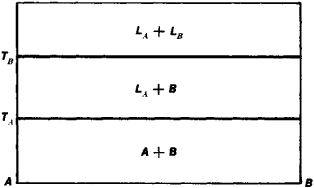Figure 1

If mutual solubility of components A and B in the liquid and solid state is nonexistent, the phase diagram (Figure 1) is represented by two horizontal straight lines drawn through points TAand TB, which correspond to the melting points of A and B. Above TB the system consists of two liquid phases LA and LB; between TB and TA, of LA and crystals of B; and below TA , of a mixture of A and B crystals. If the mutual solubility of components A and B in the liquid state is not limited but mutual solubility is nonexistent in the solid state, then upon cooling, two solid phases, crystals of A and B, precipitate from a single liquid phase L (Figure 2). The liquidus curves (geometric loci of the temperatures of the beginning of crystallization) TAEE and TBEE intersect at the eutectic point E. The liquid, the composition of which corresponds to point E, solidifies at a constant temperature to give a mixture of fine crystals of A and B. From liquids having the compositions between A and E, crystals of A start to precipitate out on cooling, with the resulting increase in the content of B in the liquid. When its composition corresponds to point E, the process is terminated by the crystallization of the eutectic. In the same way, the solidification of liquids having compositions between B and E starts with the precipitation of the crystals of B and terminates by the crystallization of the eutectic. The straight line FG drawn through point E is called the solidus curve (geometric locus of the temperatures at the end of crystallization). Solidified binary systems of this type consist of two-phase mixtures of the crystals A + B. The isotherms of the properties of such mixtures approximate the straight line AR (Figure 2).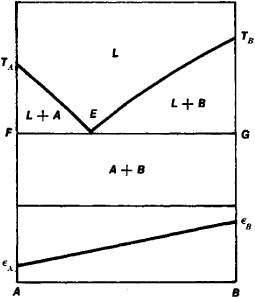Figure 2

If the components A and B possess unlimited mutual solubility in the liquid as well as in the solid state, then on cooling, only a single crystalline phase precipitates out of a single liquid phase L, namely, the solid solution 5 (Figure 3). The phase diagram of this binary system may be without a maximum and a minimum (Figure 3, I), with a maximum (Figure 3, II), or with a minimum (Figure 3, III). The isotherms of the properties have the form of continuous curves with their convex side turned upward (Figure 3, IV) or downward (Figure 3, V).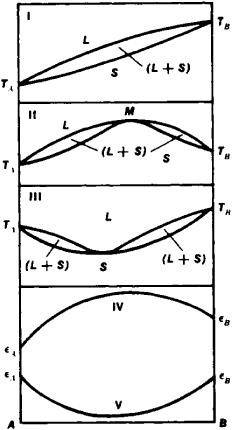Figure 3

If the mutual solubility of A and B is not limited in the liquid state but limited in the solid state, then any eutectic formed consists of a mixture of two solid solutions ɑ and β (Figure 4), in which case the limiting concentrations of the solid solutions correspond to points F and G at the eutectic temperature and to points M and N at room temperature. The composition-property isotherms (corresponding to temperature t) consists of three branches ∊Am1,n1,∊B and ∊Am2B the points m1, m2 and n1, n2 correspond to the limiting concentrations of the solid solutions ɑ and β temperature t.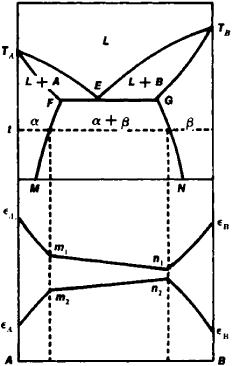Figure 4

When from the liquid L there crystallizes only a single chemical compound C, which melts without decomposition, and when solid solutions are nonexistent, the liquidus curve exhibits a rational maximum M or a singular point D, which corresponds to the composition of compound C, and two eutectic points E1 and E2, which correspond to the eutectics formed by C with A and B, respectively (Figure 5). The isotherms of properties have the form of two straight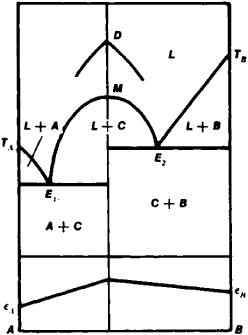Figure 5

lines intersecting at the ordinate value of compound C. More complex phase diagrams are discussed in the references listed below.

REFERENCES

Kurnakov, N. S. Izbr. trudy, vols. 1–3. Moscow, 1960–63.
Vol, A. E. Stroenie i svoistva dvoinykh metallicheskikh sistem, vols. 1–2. Moscow, 1959–62.
Hansen, M., and K. Anderko. Struktury dvoinykh splavov. Moscow, 1962. (Translated from English.)

S. A. POGODIN

References in periodicals archive ?
It is expected that there will be a good degree of H-bonding produce self-association in the pure phase in addition to mutual association in their binary systems [6, 7].
Alas, something very different awaits a star like our own when it is part of a binary system. The white dwarf component of a binary system slowly and relentlessly sips up the stellar material of its companion star.
"It's rare to see a binary system so well-aligned" said Dr.
Despite a number of investigations over the past 30 years, there remains some uncertainty about the physical nature of the CQ Cep binary system. Using the freely available eclipsing binary star model StarLight Pro (http://www.
LMA was employed for the adjustment of equilibrium data of the binary systems to obtain the parameters of Wilson's model and the equilibrium constant for each binary system.
Synthesizing of radial velocity curves of close binary systems. Astronomicheskij Zhurnal, 71(30): 420-430.
The crystal chemistry of this binary system can be largely described by octahedral units [[NbO.sub.6]] except for [Ba.sub.3][Nb.sub.2][O.sub.8], which was reported (using powder x-ray method) to adopt the salt-like palmierite-type structure with isolated tetrahedral [[NbO.sub.4]] units (85).
Astronomers have long studied binary systems in which the stars are far enough apart and bright enough to be seen as separate individuals.
A binary system could be "the ultimate cosmic 'diet plan' for heavyweight stars, which shifts over 95 per cent of their initial mass," he said.
Two other pulsars in binary systems, Ter 5 I and Ter 5 J, have highly eccentric orbits and also appear to be 60 to 70 percent more massive than the Sun (most neutron stars are only 30 to 40 percent more massive than the Sun).
Merged stars and binary systems would both be about twice the typical mass of individual stars in the cluster.
In 10 binary systems studied thus far, a "normal" dwarf or giant star tightly orbits an invisible companion object that has more than 3 solar masses--a threshold past which gravitational collapse should overcome any other force.

Site: Follow: Share:
Open / Close